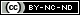## Friday, April 15, 2016Not actually Erin. & is used with permission.
PREVIOUSLY: Preventing Radiation Sickness for Dummies

We're almost done with the "Radioactivity for Dummies" series. I've talked about why ionizing radiation is bad and how to prevent it, but sometimes you can't keep it off you.

Before I can explain about doses, though, you need to know the terms that measure radiation -- and there are a lot of them.

Becquerel (Bq)
The becquerel measures rate of decay -- how often a radioactive substance releases ionizing radiation. This is known as specific activity and is why the word "active" is in "radioactive". 1 Bq equals 1 release per second per gram of substance.

This is not to be confused with counts per second, which is how often a measuring device (like a geiger counter) detects the radioactive emission. This is an important distinction; the closer to a radioactive substance a geiger counter is, the higher its counts per second will go, but the substance's rate of decay will not change.

The Becquerel does not measure how dangerous a radioactive substance is; it only measures its activity.

3.7 x 10^10 Bq = 1 curie.

Curie (Ci)
Like the Becquerel, the Curie measures the rate of decay of a radioactive substance. Unlike the Becquerel, it is a much higher number; 1 curie equals 37 billion becquerels, or 37 Gigabecquerels (GBq). This is largely due to the fact that scientific instruments were much less sensitive when it was introduced (1953) than the later Becquerel (1975).

This unit has fallen out of favor and is rarely used.

For the curious, 1 Ci is roughly equal to the specific activity of 1 gram of Radium-226 (the most common form the element).

1 Ci = 37,000,000,000 Bq.

Gray (Gy)
This unit measures absorbed dose -- in other words, how many "energy units" of ionizing radiation a certain mass of matter absorbs. 1 gray equals 1 joule of energy deposited into one kilogram (2.2 pounds) of a material.

To put that in terms you will understand, one joule (J) is the energy needed to lift one medium-sized tomato one meter off the ground. It is also the energy released when that same tomato is dropped and strikes the ground.

Since grays only measure the amount of energy absorbed and not the biological effects of it, preppers will not find much use for it. Far more useful for our purposes is the sievert, below.

This is an acronym for Radiation Absorbed Dose, and just like the Gray it measures absorbed dose. It has been supplanted by the gray, but some countries (like the USA) still use it.

1 rad = 0.01 Gy. (10 milligray, or mGy).

Another acronym, this time for Roentgen Equivalent Man. An older unit that has fallen out of favor, it has been replaced by the sievert except in the USA.

One rem equals a 0.055% chance of eventually developing cancer. 20 rems therefore equal a 1.1% chance of cancer.

1 Sv = 100 rem.
1 rem = 0.01 Sv (10 millisieverts, mSv).

1 rem = 1 rad (gamma) or (beta)
1 rem = 0.1 rad (neutron)
1 rem = 0.05 rad (alpha)

See Radiation Q factors, below, for an explanation of why rems vary.

Roentgen (R)
A largely useless unit, it measures the ionization of molecules in a mass of air by gamma or x-rays. It only describes gamma or x-rays, and only in air, which is why it is largely useless; absorbed dose is more useful to know.

That said, 1 R = 0.877 rads = 0.877 rem = 0.00877 Sv = 8.77 mSv

The good news is that you rarely need to know this information. The bad news is that if you hear it, it's likely being used to describe exposure to a nuclear detonation or an extinction-level event known as a gamma-ray burst.

Sievert (Sv)
Sieverts measure equivalent dose, which is the probability of cancer or genetic damage within a human body. The more sieverts a body absorbs, the higher the likelihood of damage to that body.

However, not all forms of ionizing radiation have the same biological effects, even if the doses are identical. To determine the equivalent dose, you multiply the absorbed dose (Gy) by a quality factor (Q) that is unique to the type of incident radiation. Gy * Q = Sv.

• Alpha radiation: Q factor 20
• Neutron radiation: Q factor 10
• X-ray, gamma, or beta radiation: Q factor 1
In other words, you're better off absorbing a dose of gamma radiation than absorbing the same dose of alpha radiation. Fortunately, alpha radiation is easy to block

1 Sv = 100 rem.

Therefore, assuming gamma rays:

1 Gy = 10 Sv = 10000 mSv = 1000 rem = 1000 rad

For non-gamma radiation, multiply by the Q factor.